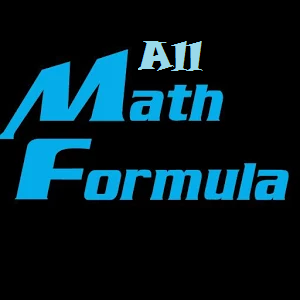## 描述

The application "All Math Formulas" is a collection of formulas out of mathematics and physics. All essentials math formula and equations: Algebra Geometry Trigonometry Calculus: Limits Derivatives Integrals

## 其他資訊

free-apps

free-apps

2015/9/29

9.25 MB

#### 支援的語言

English (United States)